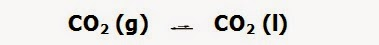Self-ionization of water - Autoionization of water - The ion product of water (kw) | Chemistry Net

# Self-ionization of water - Autoionization of water - The ion product of water (kw)

Self-ionization of water - Autoionization of water - The ion product of water (kw)

# Self-ionization of water - Autoionization of water - The ion product of water (kw)

A water molecule can act as an acid and as a base according to:

In the above reaction – autoionization of water - one water molecule acts as an acid donating a proton to the other water molecule that acts as a base – proton acceptor. From the equation we see that for every hydronium ion H3O+ that is formed a hydroxyl ion OH-  is formed also.

Therefore, at equilibrium: [H3O+] = [OH-]
The equilibrium constant kw for the above reaction is known as the ion product of water. The value of kw at room temperature is 1.007 x 10-14.

kw = [H3O+] * [OH-] / [H2O]2 = [H3O+] * [OH-] = 1.007 x 10-14     (2)

In pure water, at 25 , since [H3O+] = [OH-], equation (2) becomes:

kw = [H3O+]2 = 1.007 x 10-14  and therefore,   [H3O+] = [OH-] ≈ 10-7 M    (25 )
The pH of pure water at 25 ℃:  pH = -log [H+] = -log (10-7) =  -(-7) = 7

However, the pH of pure water would slightly decrease over time - to 6.6 – 6.8 -due to the dissolved CO2 from the air.
Carbon dioxide CO2 is slightly soluble in pure water and the solubility decreases with temperature. At pressures up to about 4 atm, the solubility follows Henry’s law:

[CO2] = kH * PCO2 = 0.032 * PCO2

The equilibrium that takes place is given in Fig. I.1:Fig. I.1: CO2 equilibrium between the gaseous and liquid phase

Once it has dissolved, a small portion of the CO2 reacts with water to form carbonic acid H2CO3 that dissociates in water to produce H3O+ decreasing the pH of the solution (Fig. I.2):Fig. I.2: CO2 reacts with water to form carbonic acid H2CO3 that dissociates in water to produce H3O+

Relevant Posts

Solving Weak Acid and Weak Base pH problems

Weak Acid Weak Base pH calculation solved example

Weak Acids and Bases - Calculate the pH of a weak acid

Polyprotic Acids / pH Calculation

Cubic Equation Calculator for Weak Acid-Base Equilibria

References

1. J.N. Butler “Ionic Equilibrium – Solubility and pH calculations”, Wiley – Interscience, 1998
2. J-L. Burgot “Ionic Equilibria in Analytical Chemistry”, Springer Science & Business Media, 2012
3. D. Harvey, “Modern Analytical Chemistry”, McGraw-Hill Companies Inc., 2000
4. J.N. Spencer et al., “Chemistry structure and dynamics”, 5th Edition, John Wiley & Sons, Inc., 2012

Key Terms

weak acids and bases, calculate the pH of a weak acid, calculate the pH of a weak base, weak acid base chemistry, weak acid base pH calculator,chemistry app calculator,weak acid base ph app calculator ,weak acid base pH chemistry applet,